# Deep Learning Made Simple [Part 1]

Published on

This series of posts was initially created as a way to explain Neural Networks and Deep Learning to my younger brother. Therefore, we are not going to assume any prior knowledge of calculus or linear algebra, among other things.

Disclaimer: The goal of this post is to be as easy to understand as possible. Because of that, some of the statements below aren't entirely accurate. Many of the larger inaccuracies are marked with *

Contents:

1. Deep Learning Made Simple [Part 1] - this post
2. Teaching a Neural Network [Part 2] (to be published)
3. ...

### What can Deep Learning do?

Deep learning is an amazing tool that can generate some very impressive results. Let's look at some of these before we dig into how it actually works.

• Deep learning can turn a photo into a painting of a particular style:• Or better yet, turn a photo into a photo of a different style: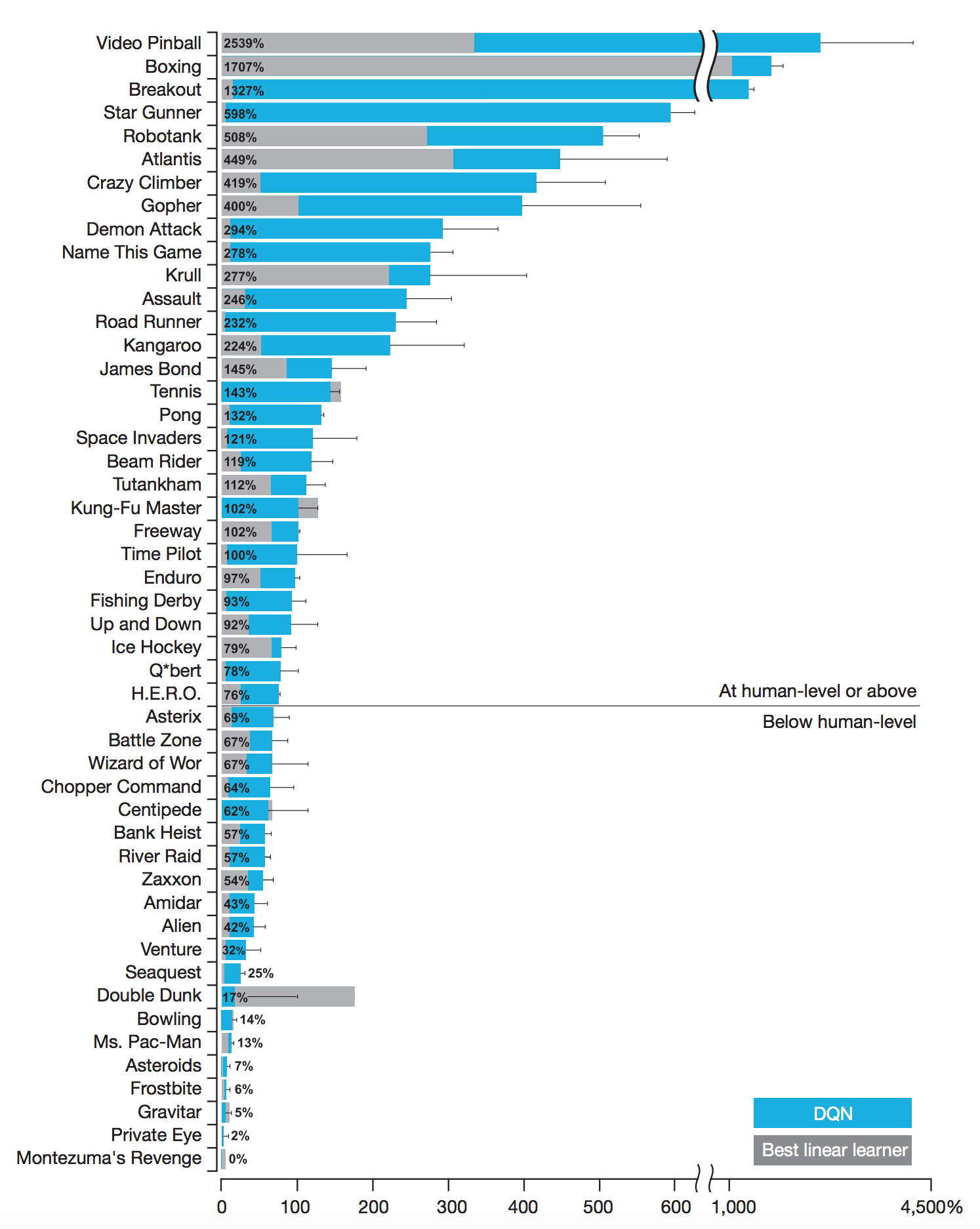• It can detect and identify objects: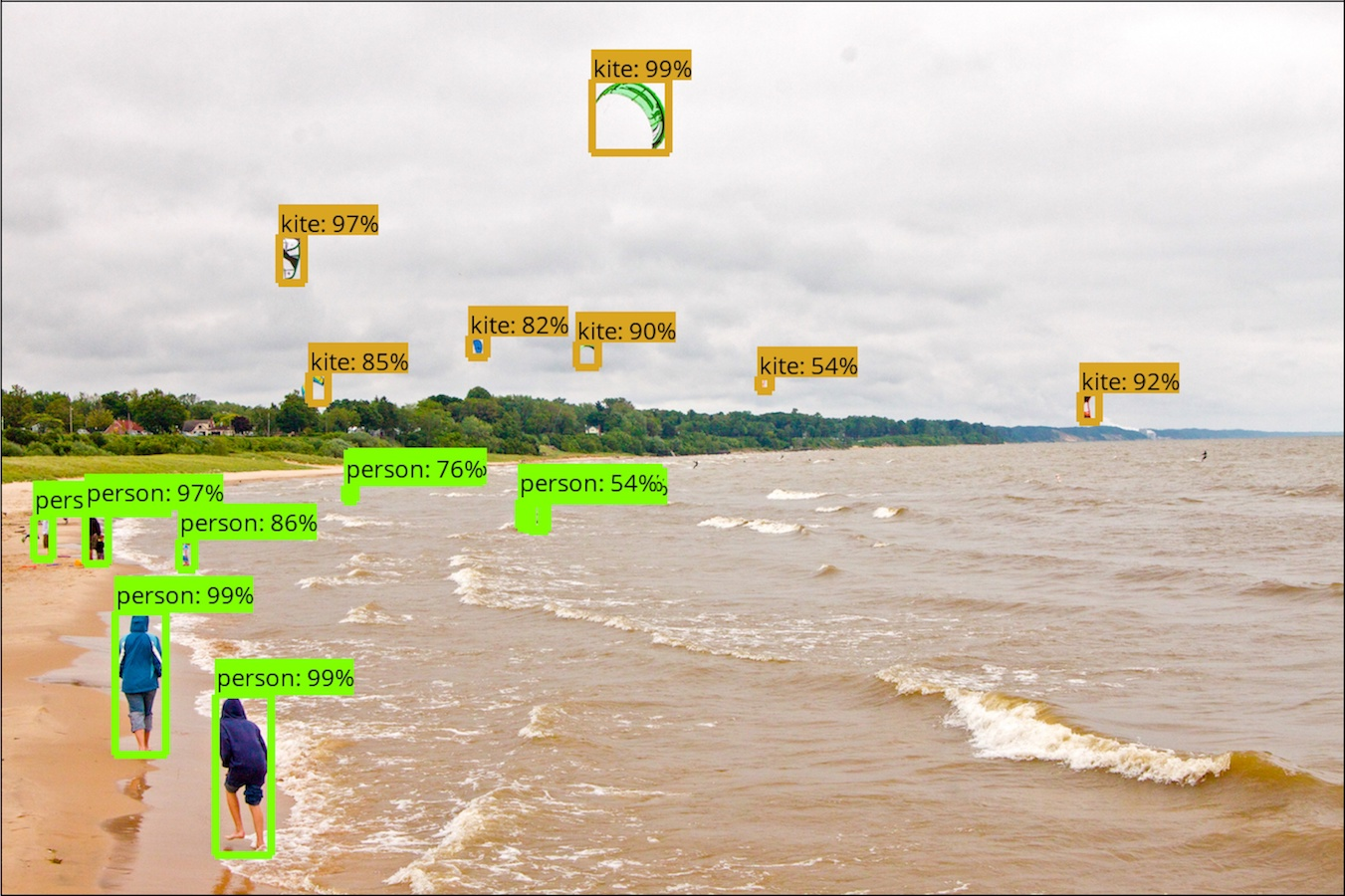• It can caption images:• Or even colorize them: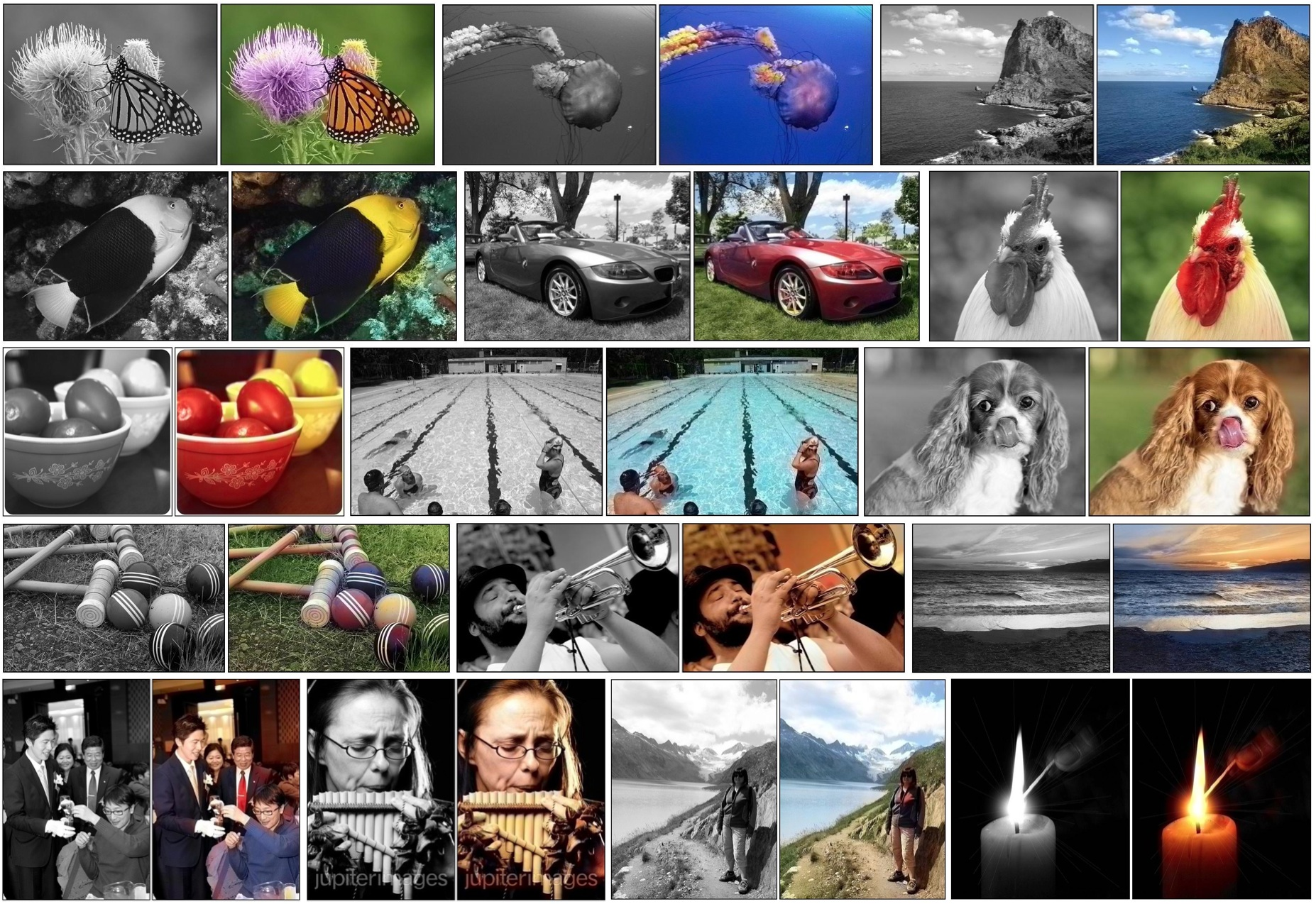• It can translate between languages
• It can recognize speech
• It can generate voices
• It can turn a drawing into a picture
• It can understand handwriting

And much much more.

### ... how?

In order to understand that, we need to dig into some basic math first. Let's define what a function is.

The definition we're going to use is as follows (from Wikipedia):

In mathematics, a function is a relation between a set of inputs and a set of permissible outputs with the property that each input is related to exactly one output.

Basically, a function takes in some number of inputs and has an output. Every time the same inputs are passed into a function, it should give us the same output.

Also note that the output of a function doesn't need to be just one number. It can also be a vector.

For our purposes, a vector is an ordered list of numbers

For example if we have a vector $v$:

• $v\_1$ is the first element
• $v\_2$ is the second element
• ...

Functions can be as complex or as simple as we want. Here are some examples:

• $y = f(x) = x + 1$
• $y = f(x) = x^2 + 1$
• $y = f(a,b,c,d) = 23 * a + 42 * b + 51 * c + d$
• items_in_picture = f(image) = ?
• speaker_identity = f(audio_signal) = ?

In the above list, think of ? as "something super complicated that we don't know how to write down."

At first glance, the last two items in the list of examples might look weird, but if you look carefully, they fit all our rules for functions:

• They relate inputs and outputs.
• For a given input, they always have the same output.

Now that we know what a function is, let's define what the purpose of deep learning is.

The end goal of most machine learning methods, including deep learning, is to figure out how to write down complex functions. In more concrete terms, machine learning methods try to estimate a function.

So how can we estimate a function we don't know how to write down? Let's look at this problem a slightly different way.

### Estimating a function

Let's pretend someone told us that there's a function $y = f(x)$ that does something interesting and they asked us to figure out what $f(x)$ is. Since they're feeling particularly generous, they'll also give us a bunch of examples of corresponding $x$ and $y$ values.

In mathematical terms, they asked us to come up with a function $g(x)$ such that $f(x) = g(x)$ for all possible $x$ values.

So how do we know if we're doing a good job? We need some way to tell how close $g(x)$ is to $f(x)$.

Let's use the distance formula that we learned in middle school (actually called the Euclidean Distance or L2 Norm). We'll call this $distance(v, w)$ where $v$ and $w$ are two vectors.

Great! So if we minimize $distance(f(x), g(x))$ for all points $x$, we should come up with the correct $g(x)$.

One slight hiccup; we don't know what $g(x)$ looks like and $f(x)$ can be "something super complicated that we don't know how to write down". How can we minimize distance if we can't write it down?

We'll tackle these problems over the next two sections.

### Taking inspiration from nature

This seems like a good time to look at the human brain. Our brain is made of 86 billion neurons. A very simple diagram of a neuron is below.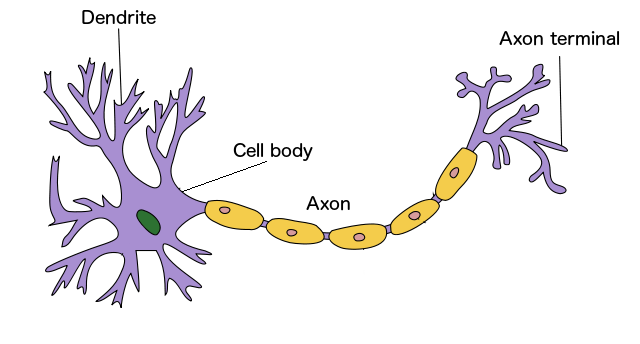The basic way a neuron works is as follows:

• Dendrites take signals from other neurons and send them to the Cell Body.
• The Cell Body takes the input signals, does some processing, and decides to "fire" or not.
• If the neuron is going to "fire," the Axon sends the output to other neurons (using the Axon terminal).

The places where the Axon of one neuron connects to a Dendrite of another neuron is called a Synapse. The human brain has between 100 trillion and 1 quadrillion synapses!

##### Artificial Neural Networks

Let's take some inspiration from the neurons in our brains and create an artificial neuron. Let's say our neuron takes 3 inputs and has one output: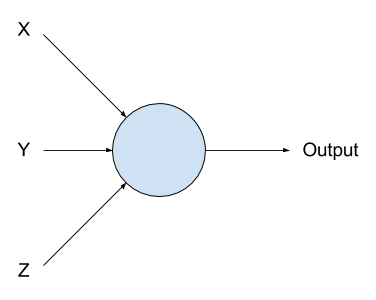So that means our neuron is a function that looks like this:

$output = f(x,y,z)$

Let's also say that our neuron is a linear function.

A linear function is a function that represents a straight line or plane.

So now we have

$output = f(x,y,z) = some\_constant * x + some\_constant * y + some\_constant * z + some\_constant$

(where all the constants can be different)

Now that we've built a neuron, let's put a bunch of these together to build a neural network!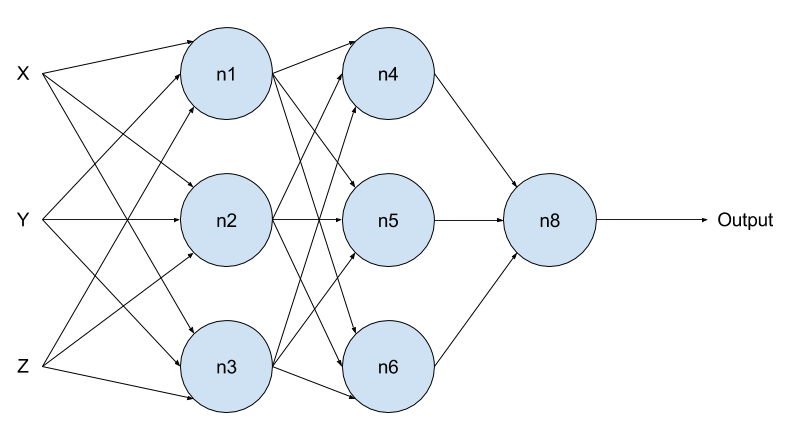Let's run through the math really quickly to see what $output$ actually is.

The output of the final layer of the neural network (n8) is:

• $network\_output = n8_{output}$
• $n8_{output} = some\_constant * n4_{output} + some\_constant * n5_{output} + some\_constant * n6_{output} + some\_constant$

The outputs of the second layer of the network (n4, n5, and n6) are:

• $n4_{output} = some\_constant * n1_{output} + some\_constant * n2_{output} + some\_constant * n3_{output} + some\_constant$
• $n5_{output} = some\_constant * n1_{output} + some\_constant * n2_{output} + some\_constant * n3_{output} + some\_constant$
• $n6_{output} = some\_constant * n1_{output} + some\_constant * n2_{output} + some\_constant * n3_{output} + some\_constant$

The outputs of the first layer of the network (n1, n2, and n3) are:

• $n1_{output} = some\_constant * x + some\_constant * y + some\_constant * z + some\_constant$
• $n2_{output} = some\_constant * x + some\_constant * y + some\_constant * z + some\_constant$
• $n3_{output} = some\_constant * x + some\_constant * y + some\_constant * z + some\_constant$

After combining all the equations above, we get:

$network\_output = some\_constant * x + some\_constant * y + some\_constant * z + some\_constant$

(again, where $some\_constant$ can be different every time it's mentioned)

So, that's a problem. Even if we have a super complicated combination of these neurons, it still can't do anything except fit a line or a plane. Why?

A linear combination of linear functions is linear.

Read that a few times and convince yourself it's true. Basically, if all you can do is add and multiply by constants, no matter how many times you do it, you'll still end up with a linear function.

So to make neural networks useful, we need to introduce nonlinearities.

A nonlinearity (or nonlinear function) is a function that does not have a linear relationship between its inputs and output. To keep it simple we'll just call these functions $nl$.

Let's change our function for each neuron to include a nonlinearity

$output = f(x,y,z) = nl(some\_constant * x + some\_constant * y + some\_constant * z + some\_constant)$

We'll dig into this more in the next post, but, for now, nonlinearities solve our problem!

In fact, now that we have nonlinearities, we can show that "for every function, there exists some neural network to represent it".* This means that any function you can come up with can be represented by a neural network!*

The proof, exact wording, and caveats of this statement are out of the scope of this post, but if you want more detail, you can look at the Universal Approximation Theorem.

Since we know $g(x)$ is a function and we know that there exists some neural network that can represent any function*, let's make $g(x)$ a neural network!

### Hot and Cold

Unfortunately, we still don't know how to minimize our distance function. Let's try to figure out how to do that.

Remember the game "Hot and Cold"? You try to find an object and as you move, your friend tells you "hotter" or "colder" depending on whether you're moving towards the target object or not.

The game looks something like this:

1. A target object is chosen
2. Start somewhere in the center of the room
3. Until you find the object:
1. You move in a direction
2. Your friend yells out "Hot" or "Cold"
3. If they yelled out "Cold," move in the opposite direction

What if we played that game to minimize a function? Let's say our "target" is to find the minimum value of our distance function.

To make things easier to write down, let's also say

$d(x) = distance(f(x), g(x))$

where $g(x)$ is our neural network and $f(x)$ is the function we're trying to estimate.

Let's rewrite how "How and Cold" is played:

1. A target value is chosen: the minimum value of $d(x)$
2. Start with a random $x$ value
3. Until you can't decrease $d(x)$ any more:
1. Move $x$ in a direction
2. Check if $d(x)$ is less than it was before
3. If $d(x)$ increased, move $x$ in the opposite direction and check again

How do we know if we can't decrease $d(x)$ any more? If we move $x$ in both possible directions and $d(x)$ increases both times, that means we found the minimum!*

#### Let's walk through that game on an example distance function.

Our random starting $x$ value is -2. So, based on the graph below, $d(x) = 5$.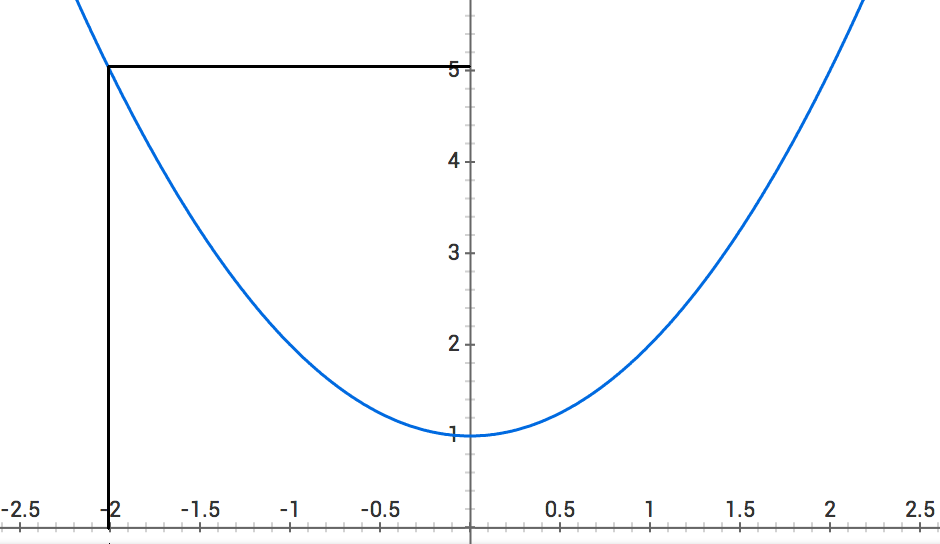Let's move to $x = -1$ and see if $d(x)$ is less than it was before.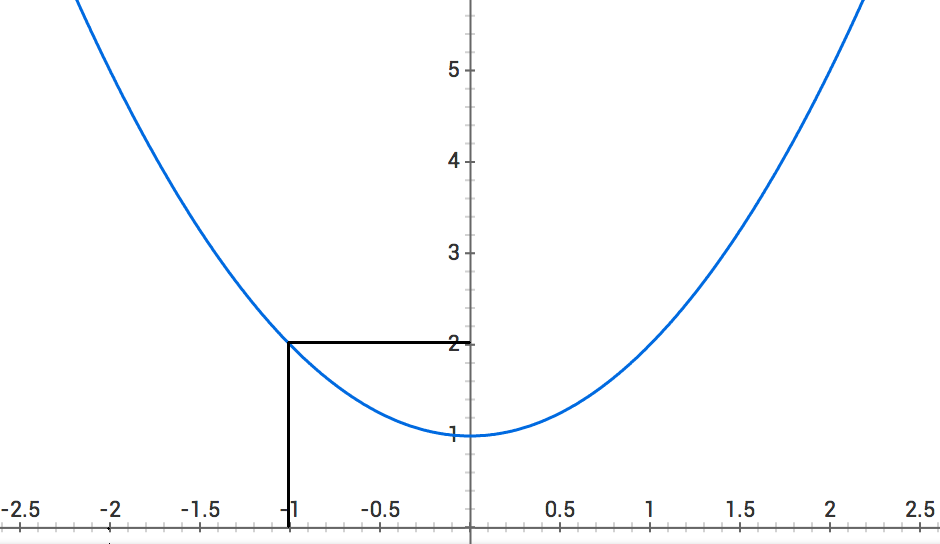$d(-1) = 2 < 5$ so we're moving in the right direction! Let's move to $x = 0$.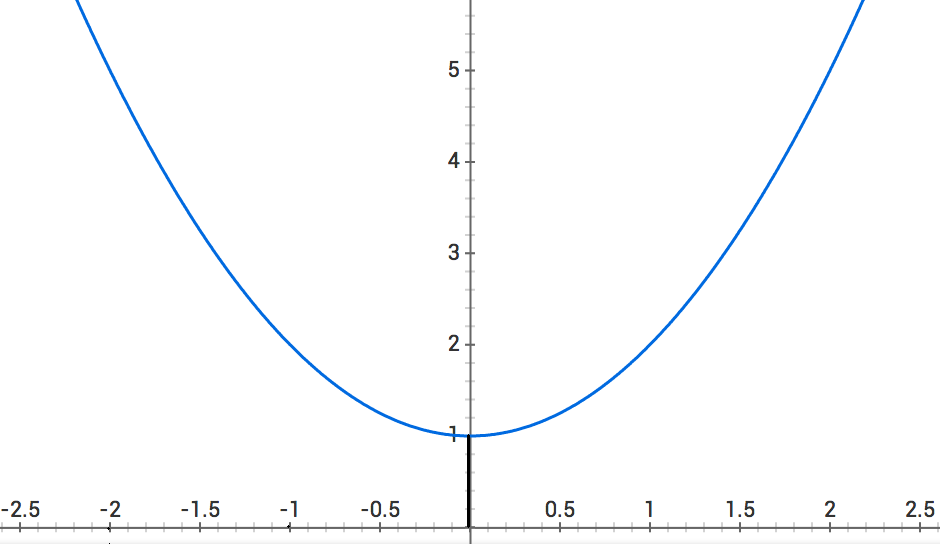$d(0) = 1 < 2$ so we're still doing a good job! Let's move to $x = 1$.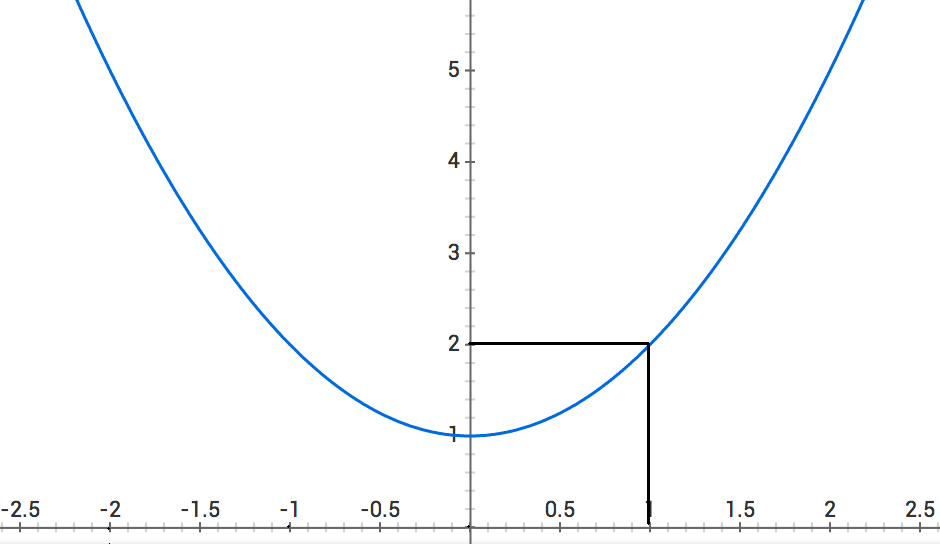$d(1) = 2 > 1$ so we're not going in the right direction. Let's do one last check at $x = -1$.$d(-1) = 2 > 1$ so looks like we found the minimum value at $d(0) = 1$!

Is there a better way to do this? We could have easily overshot the minimum point if we started at a different point or if we chose a different step size. For example, if we started at $x = -1.5$ instead of at $x = -2$, we would have never reached $x = 0$ with a step size of $1$ (we would have gone from $-1.5$ to $-0.5$ to $0.5$).

Can the slope of a line help us?

The slope of a line between two points $(x_1, y_1)$ and $(x_2, y_2)$ is $\frac{y_2 - y_1}{x_2 - x_1}$.

Therefore, the slope of $d(x)$ is defined as:

$slope_d(x_1, x_2) = \frac{d(x_2) - d(x_1)}{x_2 - x_1}$

Now let's say that $x_2 = x_1 + q$ where $q$ is some small number. Let's substitute that in:

$slope_d(x_1) = \frac{d(x_1 + q) - d(x_1)}{(x_1 + q) - x_1} = \frac{d(x_1 + q) - d(x_1)}{q}$

So if $d(x_1 + q)$ is less than $d(x_1)$, then $slope_d(x_1) < 0$ otherwise $slope_d(x_1) > 0$

With this new notation, let's change our game to the following:

1. A target value is chosen: the minimum value of $d(x)$
2. Start with a random $x$ value
3. Until you can't decrease $d(x)$ any more:
1. Subtract $slope_d(x)$ from $x$ (i.e. $x = x - slope_d(x)$)

You'll notice that the last step is the same as this:

1. If $slope_d(x) < 0$, we want to increase $x$ so we'll subtract $slope_d(x)$ from $x$ (i.e. $x = x - slope_d(x)$)
• Subtracting a negative number from $x$ will increase $x$
2. If $slope_d(x) > 0$, we want to decrease $x$ so we'll subtract $slope_d(x)$ from $x$ (i.e. $x = x - slope_d(x)$)
• Subtracting a positive number from $x$ will decrease $x$

Since we do the same thing if $slope_d(x) < 0$ or if $slope_d(x) > 0$, I collapsed them down into one case.

This version of the game also has the interesting property that the step size changes depending on the slope. As the slope gets smaller and smaller, our step size gets smaller. That means we slow down as we get closer to our target and we're less likely to overshoot the minimum!

So, now we know how to minimize a function, but the $f(x_1 + q)$ thing is a little weird, right? What if we made $q$ really really small? This is called a derivative.

The derivative of a function at a point is the slope of the function at that point.

That's pretty much all you need to know about derivatives for now. Let's look at minimizing a function that is a little more complicated.

##### Finding the minimum point on a surface

Imagine you're standing on a landscape with a bunch of hills and valleys. It's pitch black outside and you can't see anything. All you know is that you want to get to the bottom of a valley. So what do you do? You feel forward a little bit and see if the surface goes up or down. Then you feel towards the right and see if the surface goes up or down. You keep feeling around and then you finally take a step in the direction that slopes the most downwards.

This is the exact same approach we're going to take.

Let's say that we're trying to minimize some function $z = f(x,y)$: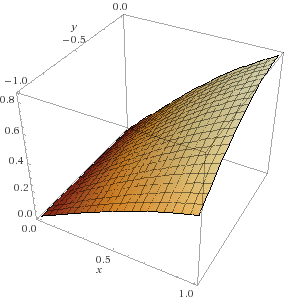3D view of $f(x,y)$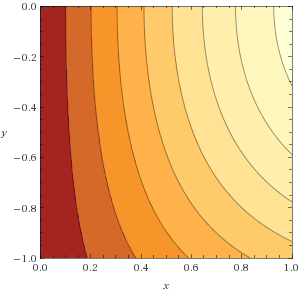Top view of $f(x,y)$. Light yellow is the largest $z$ value and dark red is the smallest $z$ value

We can't just say $slope_z(x, y)$ though, because there are multiple slopes to measure now. For example, at the point $(1,0)$, the slope in the $x$ direction (as we decrease $x$) is steeper than the slope in the $y$ direction (as we decrease $y$).

That's pretty easily solvable. Let's just say "the slope in the $y$ direction" or "the slope in the $x$ direction" instead of just "the slope". Let's write them down as follows:

• the slope in the $x$ direction of $f(x,y)$ = $slope_{z\{x\}}(x, y)$
• the slope in the $y$ direction of $f(x,y)$ = $slope_{z\{y\}}(x, y)$

These are called partial derivatives.

Let's also define one more term: the gradient. The gradient of $f(x,y)$ = $(slope_{z\{x\}}(x, y), slope_{z\{y\}}(x, y))$. Basically the gradient is a vector made up of all the partial derivatives of a function. It has a really useful property:

The gradient of a function points in the direction of the greatest rate of increase of the function

That means the negative of the gradient is the direction that slopes the most downwards. Sound familiar? That's exactly what we were doing in our pitch black landscape example!

So now our game looks like this:

1. A target value is chosen: the minimum value of $f(x,y)$
2. Start with a random $x$ value and a random $y$ value
3. Until you can't decrease $f(x,y)$ any more:
1. Compute the gradient of $f$
2. Subtract $slope_{z\{x\}}(x, y)$ from $x$ (i.e. $x = x - slope_{z\{x\}}(x, y)$)
3. Subtract $slope_{z\{y\}}(x, y)$ from $y$ (i.e. $y = y - slope_{z\{y\}}(x, y)$)

This process is called Gradient Descent and it can be used to minimize any function.*

### Putting it together

Now that we have a $g(x)$ function that can represent any other function*, a $distance$ measure to see how close we are to correct, and a way to minimize any function*, we've solved the problems that we identified at the beginning of the post!

This also means we can concretely answer the "how" question:

Deep learning uses neural networks with many layers combined with a distance function and a large list of examples to estimate a complicated function.

Let's apply this explanation to the colorization example at the beginning of the post:

• The function we're trying to estimate is $real\_color\_image = f(black\_and\_white\_image)$
• Since we don't know how to write that function down, we have a large list of corresponding $(real\_color\_image, black\_and\_white\_image)$ pairs
• Our neural network is some function $colorized\_image = g(black\_and\_white\_image)$
• (we'll look into this in more detail in a future post)
• Our distance function looks like this: $distance(real\_color\_image, colorized\_image)$

Now we can start to put all of these pieces together. Let's substitute $f$ and $g$ into our $distance$ function:

$d(black\_and\_white\_image) = distance(f(black\_and\_white\_image), g(black\_and\_white\_image))$

Since we don't have $f$, let's use one of our example image pairs instead:

$d(black\_and\_white\_image, real\_color\_image) = distance(real\_color\_image, g(black\_and\_white\_image))$

Now we just have to minimize $d$ for every example we have!

This presents a problem, however. In our "Hot and Cold" game, we modified an input to $d$ in order to minimize it, but we can't modify $black\_and\_white\_image$ or $real\_color\_image$ in the formula above. Let's rewrite $d$ to make it more clear what we need to modify:

$d(black\_and\_white\_image, real\_color\_image, g) = distance(real\_color\_image, g(black\_and\_white\_image))$

We change our neural network.

To minimize $d$, we're just playing the "Hot and Cold" game from above "in the $g$ direction"! Remember all the $some\_constant$s? We can change all of those to make the network learn!

So we start with a "random" neural network* and then slowly modify the constants until it does a good job on our examples. If we have enough examples and our network learns how to do a good job on them, it should be able to do a good job on inputs it hasn't seen before!*

This process is called training a neural network.

In the next post, we'll look into how exactly we do this. We'll also build a neural network to recognize handwritten digits!

If you want to be notified when I publish the next part, you can follow me on Twitter here.

Please feel free to comment on Hacker News or Reddit or email me if you have any questions!

If you want to learn a little more about me, check out my website or LinkedIn

While you're waiting on part 2, you can also check out my primer on load balancing at scale.

Header image from neural-style on GitHub